# Finite Element Analysis of Plate and Shell Structure

April 1, 2018

The plate and shell structure is a structure in which the dimension in the thickness direction is much smaller than the size in the length and width directions. Among them, the straight surface is called a plate, and the curved surface is called the shell. Because the shell considers the surface curvature, it is theoretically much more complicated than the plate. At the same time, the plate as a special case of the shell. In the practical analysis, the plate can be replaced by the shell element.

## 1. Shell Structures

The shell structure is widely used in automobile, ships, and aerospace fields because of its excellent lightweight and easy-manufacturing properties. The bodies of the car, aircraft, submarine, and pressure vessels belong to shell structure as shown in the figures below.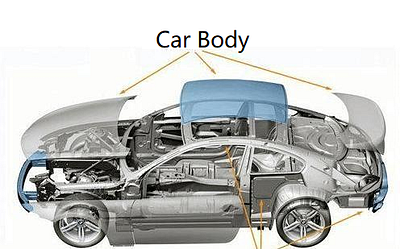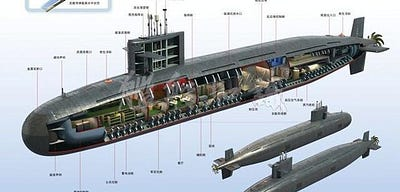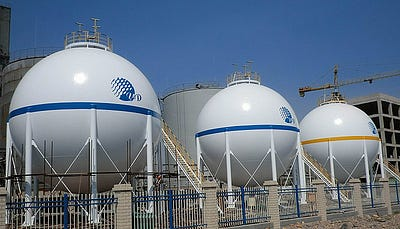## 2. Shell Theory

The shell theory is based on the elasticity mechanics and some engineering assumptions (such as Kirchhoff hypothesis, Kirchhoff-Love assumption, etc.), and studies the stress distribution, deformation, and stability of shell structures under external forces. The shell theory is a more complex theory in engineering mechanics.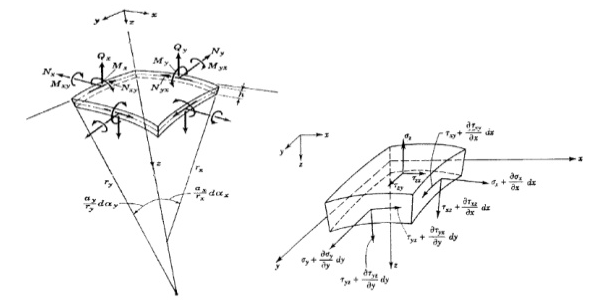## 3. Finite Element Analysis

For the plate and shell structures, WELSIM offers efficient solutions to evaluate the characteristics quickly. Today we take a simple case to understand the supported features provided by WELSIM.

### 3.1 CAD Model Creation and Import

In WELSIM, a simple plate geometry model can be created. The graphical interface is as shown in the figure: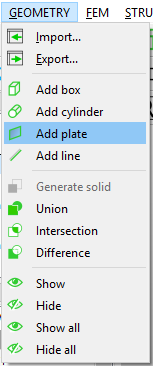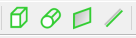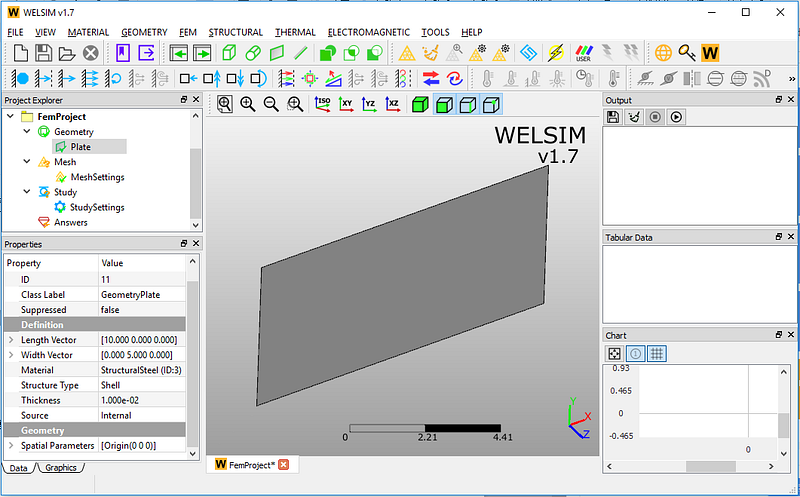You can also import a STEP-format CAD file that contains complex surfaces. Importing a complex surface model is shown below.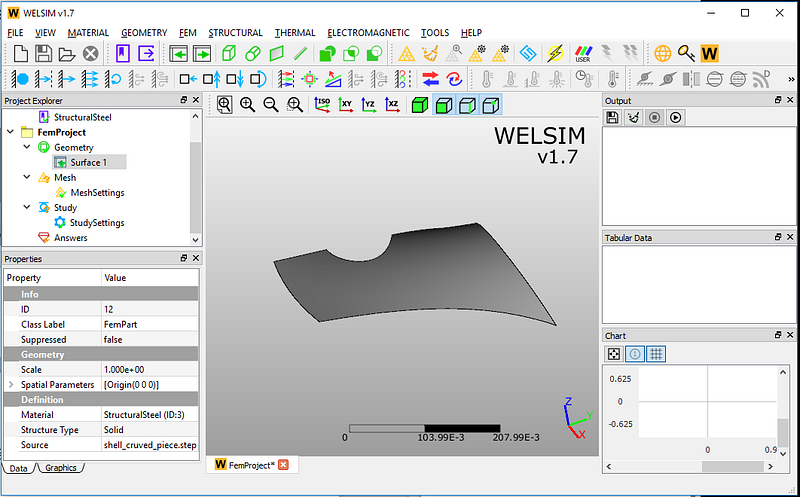For the imported models, the system needs to know the type of the structure. We will change the Structure Type from Solid to Shell in the Properties window.As the structure type is set to Shell, there will be new properties of thickness and integration points, and the user can define the thickness of the shell.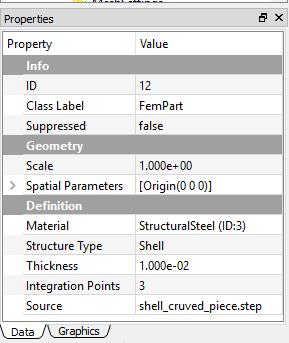### 3.2 Mesh Generation

The current shell solver supports TRI3 element, so we mesh the domain using linear triangle element. Simply set the parameters, and you’ll quickly generate the mesh. There are 263 nodes and 437 triangular elements created in the mesh: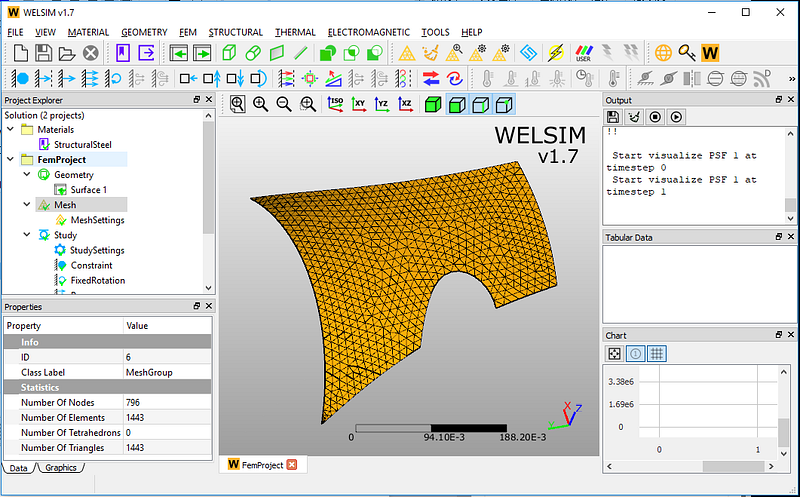### 3.3 Static Analysis

For the static analysis of the shell, the boundary and body conditions currently supported by WELSIM are fixed support, fixed rotation, displacement, force, pressure, body force, acceleration, standard Earth gravity, and rotational velocities. Among them, the fixed rotation is specifically for the shell structure.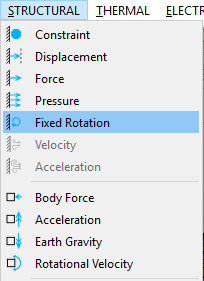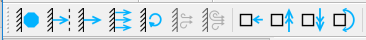Here we apply fixed support and rotation to one edge.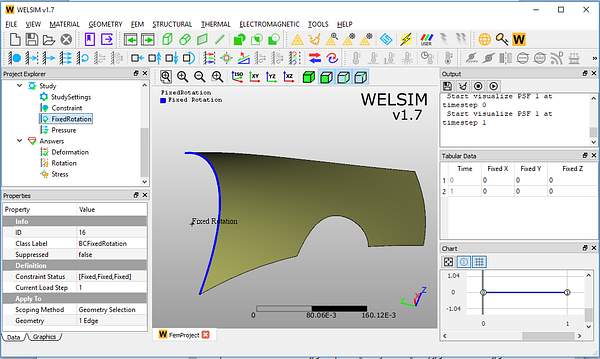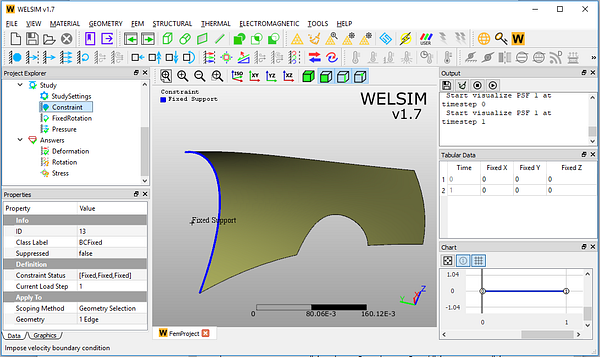Next, we impose a pressure boundary condition with the magnitude of 1e3 on the shell surface.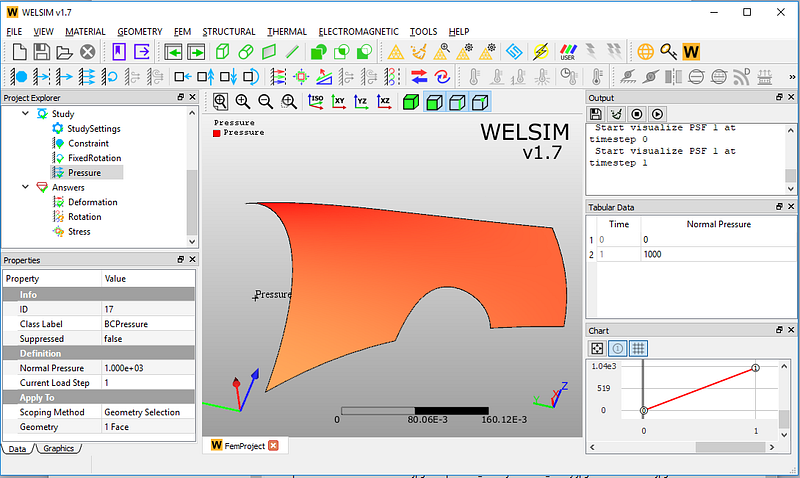The results of the shell analysis supports displacement, rotation, stress, strain, reaction forces, and reaction moment. Among them, the rotation and reaction moment are specifically for the shell structure.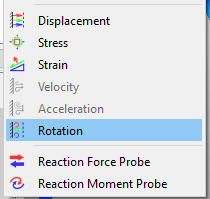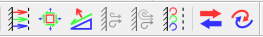The result of total displacement. The maximum magnitude is 2.997e-5.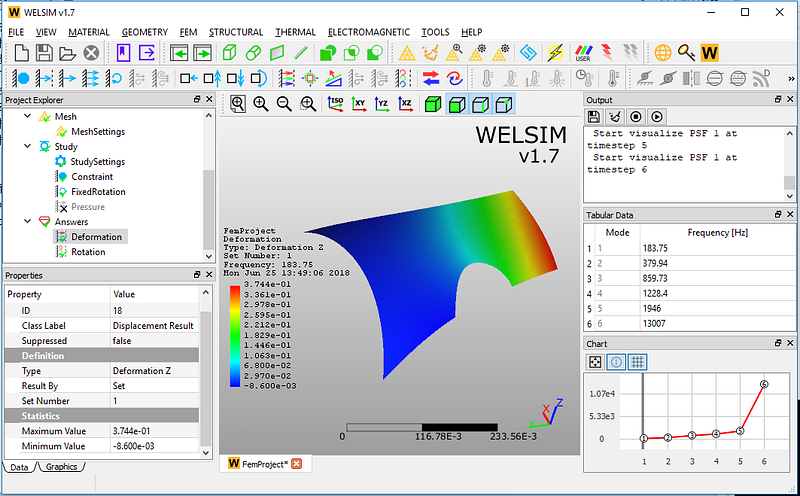The result of the total rotation. The maximum magnitude is 1.797e-4 rad.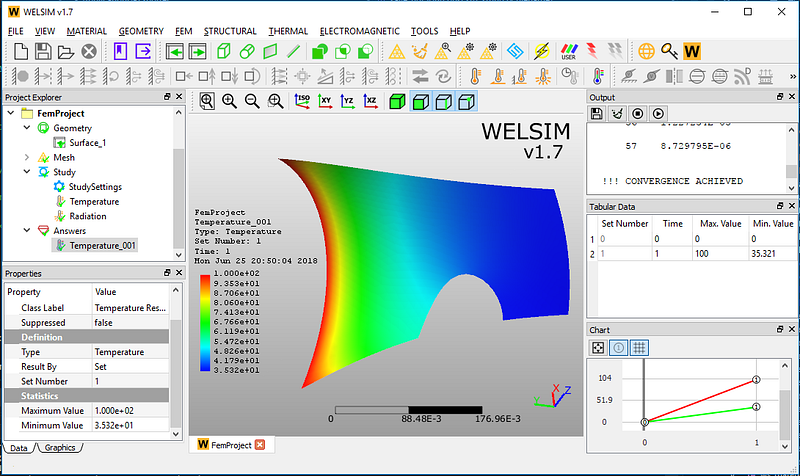The von-Mises stress result shows here as well. The maximum stress is 3.861e6. It can be seen that the stress concentration happens around the location of the curved areas.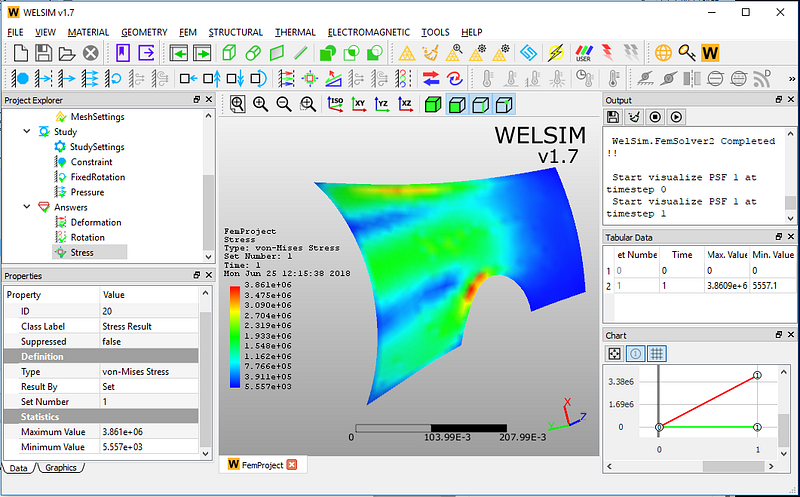### 3.4 Modal Analysis

Modal analysis is a method to study the dynamic characteristics of structures, and it is the application of system identification methods in the field of vibration engineering. The vibration mode is an inherent and integral characteristic of the elastic structure. Through the modal analysis method, the characteristics of the primary modes of the structure in a certain vulnerable frequency range are discovered, and it is possible to predict the actual vibration modes of the structure under the influence of the external or internal force. Therefore, modal analysis is an essential method for structural dynamic design and equipment fault diagnosis. Modal analysis is used to determine the fixed frequency of components and assemblies and is the starting point of dynamic analysis. Here, our modal analysis can determine the natural frequency and mode shape of a shell structure.

Similar to the static analysis, we fix the displacement and rotation of the shell on one edge.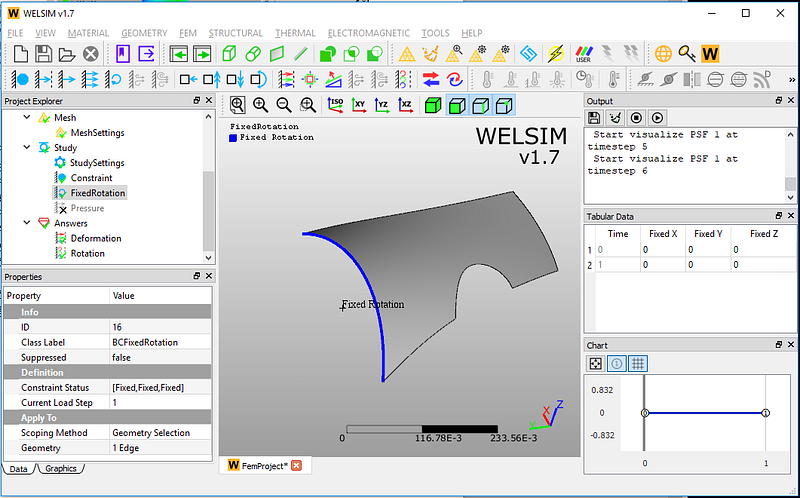The natural frequency is the vibration frequency of the object when the workpiece is constrained. In the modal analysis, WELSIM uses the Lanczos method to solve the eigen-value problems. Here we set the number of modes to 6. After calculation, you can see the results of the natural frequencies as shown.

In the shell structure analysis, the natural frequencies of the first six modes are 183.75, 379.94, 859.73, 1228.4, 1946, and 13007 Hz respectively. At the same time, the relative deformation results are given here.### 3.5 Thermal Analysis

As the solid structure, we can conduct the thermal studies on the plate and shell structures using WELSIM. In this thermal analysis example, we impose two types of thermal boundary conditions.

A fixed temperature is imposed onto the curved edge with the magnitude of 100 degrees.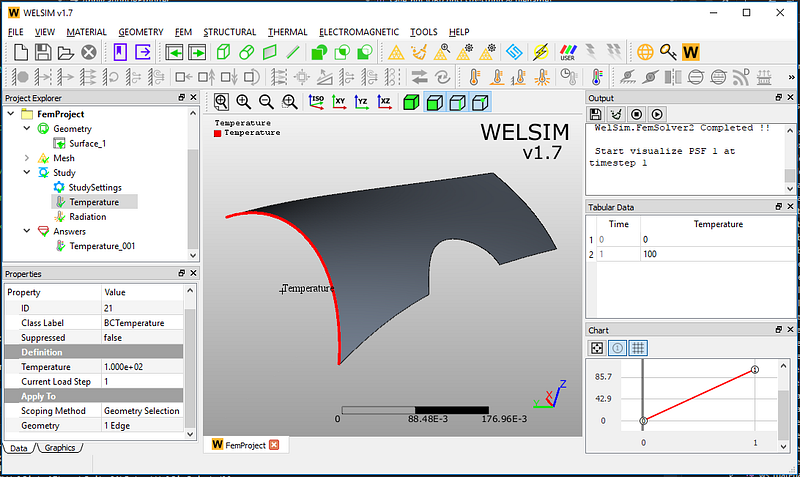Next, we impose the heat radiation boundary condition on the surface of the shell body, the radiation coefficient is 1e-4, and the ambient temperature is set to 23 degrees.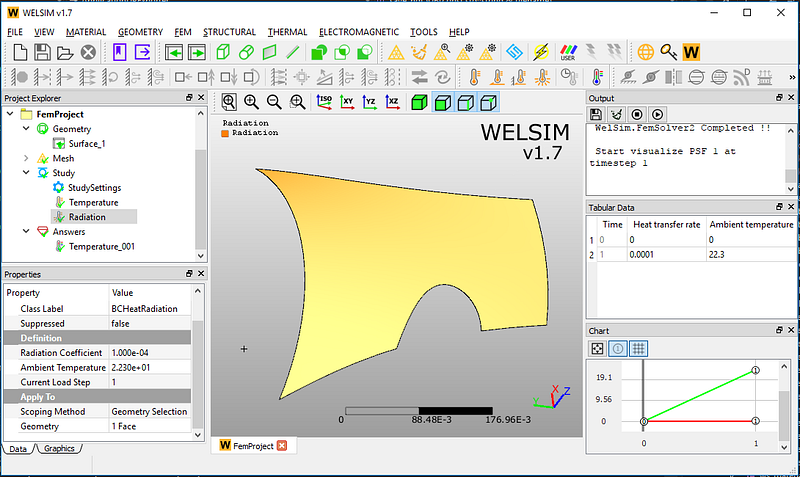Solving the model, we can quickly get the temperature distribution on the shell structure. The minimum value is 35.32, and the maximum value is 100.### 4. Summary

In this article, we conduct the static, modal, and thermal analyses for the shell structure using WELSIM. The process is quick, and the solution is accurate. Through such type of finite element analysis, it saves time in understanding the shell structure at various working environments. The numerical solution also provides critical guidelines for the structural optimization.

Note: This example is only used as a software demonstration, not as a reference for actual engineering analysis. Shell analysis is a new feature introduced in WELSIM v1.7.